Trial ends in
JoVE Science Education
Chemical Engineering

A subscription to JoVE is required to view this content.
You will only be able to see the first 20 seconds.

Viscosity of Propylene Glycol Solutions
Science Education (Chemical Engineering)
Kinetics of Addition Polymerization to Polydimethylsiloxane
Science Education (Chemical Engineering)
Vapor-liquid Equilibrium

Viscosity of Propylene Glycol Solutions

Overview

Source: Michael G. Benton and Kerry M. Dooley, Department of Chemical Engineering, Louisiana State University, Baton Rouge, LA

Viscosity is a measure of a fluid's resistance to flow, and it is a useful parameter in the design of efficient product processing and quality control in a wide range of industries. A variety of viscometers are used to obtain the most accurate readings of experimental materials. The standard method of measuring viscosity is through a glass tube viscometer, which estimates viscosity by measuring the amount of time it takes fluid to flow through a capillary tube made of glass1.

Rotational viscometers operate by applying shearing forces and measuring the time it takes a flowing1. These viscometers make use of the flowing force of the fluid, and they can use either a spring system or a digital encoder system1. Different measuring systems exist as well, with the standard being a cone and plate system, where fluid flows under the cone shape and over the plate, in order to minimize shear stress1. Parallel plate systems use two parallel plates and is ideal for measuring across temperature gradients, allowing a smooth transition1. Couette systems use a cup and filling material, and the fluid flows in between the two1. These systems are best for materials with low viscosity, since this system minimizes shear stress, but the system is also harder to operate routinely due to issues with cleaning and needing larger volumes of fluid1.

In this experiment, a Cannon-Fenske viscometer will be used to measure the viscosities of several propylene glycol solutions to determine the relationship between viscosity and composition.

Principles

Kinematic viscosity is the ratio of dynamic viscosity to density. The ratio of shear stress to the shear rate is the dynamic viscosity of a fluid, which is a measure of the resistance to deformation in laminar flow for a Newtonian fluid. This ratio is unique to kinematic viscosity, which enables the interpolation for an unknown variable by considering two variables, kinematic viscosity and concentration.

Temperature, density, and composition can create changes in the deformation of the flow. Pressure is negligible on liquid-phase fluids, so it does not factor into this experiment. Kinematic viscosity can be measured using a viscometer because of the relationship presented in the Hagen-Poiseuille law, which expresses that the pressure drop is due to gravity, dynamic viscosity, and kinematic viscosity. Kinematic viscosity can also be related to time using a viscometer constant specific to each capillary glass,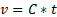where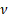is the kinematic viscosity, C is the viscometer constant, and t is time. Viscometer constants are generally measured and provided by the manufacturer of the viscometer. The above equation is used to determine kinematic viscosity, which can be converted to dynamic viscosity by multiplying by the density of the fluid,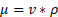where µ is the dynamic viscosity,is the kinematic viscosity, and ρ is the density. This information can be used to relate the concentration of solute and dynamic viscosity graphically.

The Cannon-Fenske Viscometer is widely used to measure the viscosity of various solutions. The viscometer is immersed in a large water bath that contains a temperature control coil and fin to maintain constant thermoequilibrium. The capillary constricts the downward flow of the liquid, so that the time required for the liquid to travel through the capillary region is multiplied by the viscometer constant to give the kinematic viscosity. Larger capillary glassware is used to measure solutions with higher viscosities. More viscous solutions take longer to travel through a capillary glass. This information can be used to compare the unknown to known concentrations.

Procedure

1. Preparing the Viscometer

1. Prepare seven solutions with varying concentrations of propylene glycol in water (between 0 - 100 mole % polypropylene glycol). Label all solutions. These will be used for calibration. Obtain a sample of the unknown concentration and set it aside
2. Check the samples for lint, dust, or other solid material. If needed, filter the sample through a sintered glass filter or fine mesh screen.
3. Clean the appropriate viscometer using water, and dry with filtered air to remove the final traces of solvents. Lower viscosity solutions will use size 50 capillary glassware, size 100 will be used for the unknown solution, and higher viscosity solutions will use size 150 capillary glassware.

2. Charging the viscometer

1. Pour the sample into the viscometer until it fills at least half of the bigger bulb. Then, wipe the arm clean.
2. Place the viscometer into the holder and insert it into the constant temperature bath. Align it vertically with a small plumb bob in the large tube or use a self-aligning holder.
3. Allow the sample to equilibrate to the water bath temperature. Allow approximately 10 min for the sample to come to the bath temperature at 40 °C and 15 min at 100 °C.
4. Apply suction to the arm and draw the liquid into the tube.

3. Measuring the Efflux

1. Use a stopwatch and measure the time required for the meniscus of the sample to flow between the indicated markings. This is the efflux time
2. Repeat steps 6 and 7 for duplicate runs. And repeat the entire procedure for each sample.

Viscosity is a measure of a fluid's resistance to flow and it is a useful parameter in the design of efficient product processing and quality control in a wide range of industries. Viscosity describes the internal friction of a moving fluid. A fluid with a high viscosity resists flow, whereas a fluid with a low viscosity flows more easily. A glass tube viscometer is commonly used to determine viscosity, which measures the time it takes for a fluid to flow through a capillary tube. Rotational viscometers such as with a parallel plate geometry, apply shearing forces and measure the torque required to rotate the fluid. This video illustrates the principles of viscosity, demonstrates how to use a viscometer to determine the unknown concentration of a propylene glycol solution, and discusses some industrial applications.

To begin, let's review the principles of viscosity. The dynamic viscosity of a fluid describes the force per unit surface area, known as the shear stress, that is required to move fluid, in this case, parallel to a plane. The fluid in close contact with the bottom plate stays at rest, and the fluid touching the top plate is the same speed as that plate. This velocity gradient is due to internal friction between layers of fluid, and is known as the rate of shear deformation. The dynamic viscosity is defined as the shear stress divided by the rate of shear deformation. The viscosity can be influenced by temperature, fluid density, and composition. Pressure changes, however, have a negligible effect. Once the dynamic viscosity is known, the kinematic viscosity is the ratio of dynamic viscosity to the fluid's density. This is a measure of the fluid's resistance to flow due to gravity. Several instruments measure kinematic viscosity experimentally, such as the Canon-Fenske viscometer. This apparatus consists of a large temperature controlled water bath with fins to maintain constant thermo equilibrium. Different sized capillary tubes are used depending on the approximate fluid's viscosity. Using this apparatus, fluid is poured into the tube and suctioned past the etched markings. Then, the time required for the fluid to travel back through the capillary tube is measured, known as the efflux time. The efflux time is then multiplied by the viscometer calibration constant, which is specific to the capillary glass used to give the kinematic viscosity. Now that you know the fundamentals of viscosity, let's measure the kinematic viscosity of known propylene glycol solutions to determine the concentration of an unknown sample.

Start the experiment by preparing seven solutions for calibration with varying concentrations of propylene glycol and water. Label all solutions with the concentration. Obtain a sample of the unknown concentration, and set it aside. Check all liquid samples for lint, dust, or solid particles before using, and if necessary, filter the sample through a centered glass filter. Next, clean the viscometer using water and dry it with filtered air. Remember to use the appropriate size glass capillary tube for the viscometer, which is dependent on the approximate viscosity of the solution to be tested.

Now, pour a sample into the viscometer, then wipe the arm clean. Next, place the viscometer into the holder and insert it into the temperature bath. Wait 10 to 15 minutes to allow the sample to equilibrate to the bath temperature. Then apply suction to the arm, and draw the sample into the tube. Now take a stopwatch and measure the time required for the sample to flow freely down the capillary tube after leaving the marked end point. This is your efflux time. Repeat the procedure for each sample. Now that all of the viscosities have been measured, let's take a look at the results and determine the concentration of the unknown sample.

To find the concentration of the unknown sample use the data of the known samples and interpolate the viscosity of the unknown solution. First, determine the kinematic viscosities by multiplying the measured time by the viscometer constant. Then convert the kinematic viscosity to dynamic viscosity by multiplying by the mass density. Next, plot the kinematic viscosities as a function of the molarities of the known samples. The graph shows that viscosity increases with increasing propylene glycol concentration. Determine the linear relationship between kinematic viscosity and propylene concentration. Then, interpolate the concentration of the unknown sample using its measured viscosity. In this demonstration, the unknown sample contained 45 mol percent of propylene glycol. Now that you have learned how the viscosity is used to determine concentration, let's examine the many applications of viscosity in industrial settings.

Testing viscosity is important in many industrial fields ranging from petroleum refinery and solar cell production to biomedical applications and research. The precise measurement of viscosity in the petrochemical industry is of high priority because it is used not only to determine crude oil composition, but also to evaluate quality control in petrochemical products. Viscosity varies from crude oil to liquid hydrocarbons, and it must be considered for efficient extraction, handling, and refinery operational conditions. Rheological characterization of highly concentrated suspensions is also mandatory for product development of applications involving complex fluids, such as those used in the metalization of solar cells. The flow properties of conductive pastes have to be carefully adjusted to guarantee undisturbed, defect-free processing and prevent sedimentation and particle aggregation. In medicine, micro rheological techniques are diagnostic tools that investigate red blood cell deformability, and detect blood diseases such as sickle cell anemia, which affect blood viscosity. Protocols utilizing micro fluidics with adjustable shear rates and image processing are utilized to quantitatively determine red blood cell aggregation in microcirculation. These protocols are also used to characterize human tissue, such as brain matter, to better understand structure and engineer bio inspired materials.

You've just watched JOVE's introduction to viscosity. You should now know the fundamental principles of viscosity, be able to operate a viscometer, and understand the importance of viscosity in a variety of applications. Thanks for watching.

Results

In this experiment, the viscosity of several concentrations of propylene glycol were measured. As expected, the viscosity was found to increase with propylene glycol concentration. The time for the sample solutions to traverse the viscometer were measured and used to determine the kinematic viscosity. Numerous measurements were collected to minimize random error.

The kinematic viscosity was determined using the time as measured and the viscometer constant: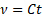Then, the kinematic viscosity was multiplied by the density to give the dynamic viscosity: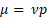The unknown concentration was calculated and compared to the known sample solutions. Linear interpolation was used to estimate the concentration, and the relationship was best fit to a linear function (Figure 1).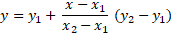For the above equation, two known data points of kinematic viscosity and concentration were used, and x was set as the measured viscosity of the unknown solution. The solution was solved for y to find the concentration of the unknown sample. The Excel graphing feature can also be used to plot a trendline through the data set and give an equation of best fit.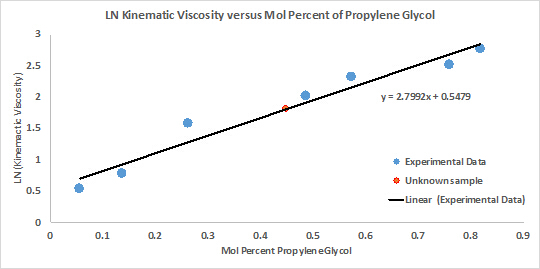Figure 1: The relationship between concentration of solution and viscosity demonstrated a linear fit.

This graph shows that the natural logarithm of viscosity and composition follow a linear relationship. As solute composition increases in a solution, viscosity also increases. Knowing this relationship, the concentration of the unknown solution is easily found by measuring its viscosity and relating it to the known relationship between concentration and viscosity. The experimental accuracy can be improved by measuring more known concentrations or using a more precise thermometer.

Applications and SummaryX